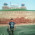Pranay Rana: Expression Tree

## Monday, April 16, 2012

### Expression Tree

What is Expression Tree ?
Expression is not like other code, but it like binary tree data structure where each node is representing an object children(s). Expression tree is get derived from the Lambda Expression which you can see easily once you can drill down in framework class. One good reason of Expression tree is it allows to create dynamic linq queries.

Expression Class
```using System.Collections.ObjectModel;

namespace System.Linq.Expressions
{
// Summary:
//     Represents a strongly typed lambda expression as a data structure in the
//     form of an expression tree. This class cannot be inherited.
//
// Type parameters:
//   TDelegate:
//     The type of the delegate that the System.Linq.Expressions.Expression
//     represents.
public sealed class Expression : LambdaExpression
{
// Summary:
//     Compiles the lambda expression described by the expression tree into executable
//     code.
//
// Returns:
//     A delegate of type TDelegate that represents the lambda expression described
//     by the System.Linq.Expressions.Expression.
public TDelegate Compile();
}
}
```
Syntax of Expression
Expression<Func<type,returnType>> = (param) => lamdaexpresion;
NameSpace System.Linq.Expressions; contains defincation of Expression Tree class. so you need to include this namespace before using the Expression in your code.
Example
```Expression<Func<int,int, int>> lambda = (a,b) => a *  b;
```

Expression Tree for the above expression

Install Expression Tree visualizer
If you are not having visualizer to view expression tree than you can install it by following below instruction
Compile code : Official_Visual_Studio_2008_C#_Samples\ExpressionTreeVisualizer\C#\ExpressionTreeVisualizer\
Get Dll from:
bin\Debug\expressionTreeVisualizer.dll
Install Dll on path : C:\Program Files\Microsoft Visual Studio 9.0\Common7\Packages\Debugger\Visualizers

Expression Tree Parts
Expression tree is consistence of following part, each part is shown in blow images.
Body

Parameters

NodeType and Type Of Expression

Difference Between Lamdab Expression and Expression
In above example I assigned lambda expression to Expression type, by doing that way enviroment represent lambda expression as expression tree not as lambda expression. And if you see memory represtation of expression tee its a object represention of expression which is already seen in expression tree visualizer, which is differnt than lambda epxssion IL.
when you write
```Func<int,int, int> lambdaExpression = (a,b) => a *  b;
```
its a lambda expression.

Important point to Note here is
You cannot use lambda expressions with a statement to create an expression tree
```Expression<Func<int, int, bool>> IsAGreater =
(a, b) => { if (a > b)  return true; else return false; };
```
above code throw compile time error.

How to Create Expression Tree ?
Fist Way:
First way is very easy that I already sawn is above discussion "Create Lambda expression and assign it to expression."
```Expression<Func<int,int, int>> lambda = (a,b) => a *  b;
```
Something as above.

Second Way:
To create expression you need to follow the below steps one by one. I am also going to show the functions that can be used in dynamic expression.
```private void CreateExpressionTree()
{
```
Create parameter for the expression.
```ParameterExpression exp1 = Expression.Parameter(typeof(int), "a");
ParameterExpression exp2 = Expression.Parameter(typeof(int), "b");
```
Create body of the expression, over here in this example using multiply function to get the multiplication of the two parameter.
```BinaryExpression exp = Expression.Multiply(exp1,exp2);
var lamExp = Expression.Lambda<Func<int, int, int>>
(exp, new ParameterExpression[] { exp1, exp2 });
```
Compile method compile the expression tree and Invoke allows to execute the compiled expression tree. As you see you need to pass the values for the parameter of expression tree.
```int c = (lamExp.Compile()).Invoke(2,5);
Response.Write(c);
}
```

Application of Expression Tree ?
One Application I fond for the Expression tree is to make use of expression to build the dynamic linq query.
Example 1 : - Bind query to get data and sort the data dynamically. As you see in below code I build query and sorting data by passing name of the property in people object. In example Email property used to sort which can be replace by Name property.
```private void BindAndSort()
{
List<people> people = new List<people>
{
new People(){ Name = "Pranay",Email="pranay@test.com",CityID=2 },
new People(){ Name = "Heamng",Email="Hemang@test.com",CityID=1 },
new People(){ Name = "Hiral" ,Email="Hiral@test.com",CityID=2},
new People(){ Name = "Maitri",Email="Maitri@test.com",CityID=1 }
};

ParameterExpression param = Expression.Parameter(typeof(People), "People");

Expression ex = Expression.Property(param, "Email");

var sort= Expression.Lambda<Func<People, object>>(ex, param);

var sortedData =
(from s in people
select s).OrderBy<people, object>(sort.Compile()).ToList<people>();
GridViewNotional.DataSource = sortedData;
GridViewNotional.DataBind();
}
```
Example 2 : - Build query to search data from the people entity using Email property and Email starts with "h". Over here property can be replace with the Name property.
```ParameterExpression param = Expression.Parameter(typeof(People), "People");
Expression ex = Expression.Property(param, "Email");

// Get the method information for the String.StartsWith() method
MethodInfo mi = typeof(String).
GetMethod("StartsWith", new Type[] { typeof(String) });
MethodCallExpression startsWith =
Expression.Call(ex, mi, Expression.Constant("h"));

Expression<Func<People, bool>> expression =
Expression.Lambda<Func<People, bool>>(startsWith, param);

var searchData = (from s in people
select s).Where(expression.Compile()).ToList();
```
Both the Example 1 and Example 2 methods can be replace with the generics.

1.2.3.